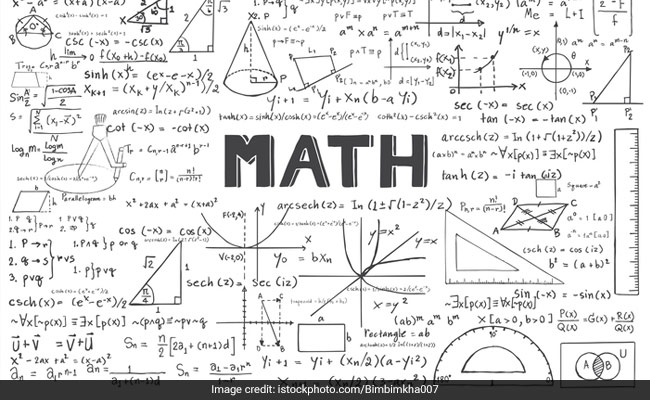# Basic Vs Standard: CBSE Class 10 Mathematics Paper Marking Scheme ExplainedCBSE will hold class 10 mathematics exam on March 12

New Delhi:

CBSE board exams are due to start this week. The exams would begin with skill-based subjects and then proceed to main subjects. A litmus test for class 10 students will be the exam for Mathematics.

2020 is the first year when CBSE will hold exam for not one but two mathematics papers for class 10 students.

In the beginning of 2019, CBSE announced that it will introduce two-levels of Mathematics for class 10 students – Basic, and Standard. The idea behind introducing two different papers was to cater to the two sets of students; one who wish to take up mathematics after class 10 and the other that doesn’t. Basic Mathematics paper was introduced for the second set.

The Mathematics exam is scheduled on March 12. Exam for both levels will be held on the same day.

What is the difference between the two papers? In simple terms, Basic Mathematics is supposed to be easier than Standard Mathematics. But that is not the only case.

The difference in the two papers is not limited to the difficulty-level of the paper. As the marking scheme and curriculum explain, the weightage allocated to different components of the curriculum also varies between Basic and Standard Mathematics.

In case of Basic Mathematics, 32 marks are allotted to questions which require remembering facts, terms, concepts and answers. In case of Standard mathematics, 20 marks’ worth of questions will require remembering facts, terms and concepts.

Similar difference is present for other components. In Basic Mathematics paper, 28 marks are allotted for questions which would demonstrate a student’s understanding of concepts. In Standard mathematics paper, it is worth 23 marks.

Application based questions will carry 12 marks in basic Mathematics paper and 19 marks in Standard Mathematics paper. and last but not the least, Questions which require analyzing, evaluation and compiling of information will carry 8 marks in Basic Maths paper and 18 marks in Standard Maths paper.